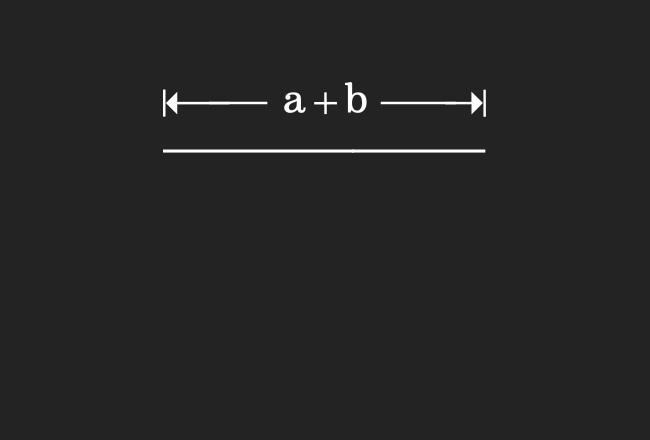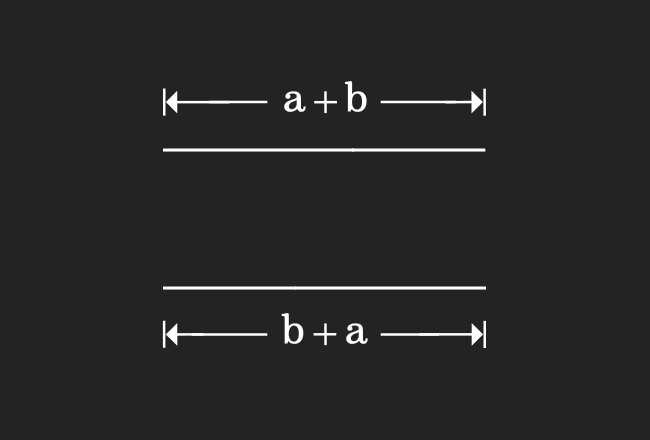# Proof of Commutative property of Addition

The commutative law of addition can be derived in algebraic form by the geometrical approach. In this method, the lengths of line segments are expressed in algebraic form and they are joined geometrically for proving the commutative rule of addition in algebraic form.

### Calculate the Length of a Line segment

We are going to draw a line segment geometrically in this step by connecting two line segments.1. Draw a line segment and its length is taken as $a$ units.
2. Draw another line segment and its length is taken as $b$ units.
3. Join the first line segment with second line segment and it forms a new line segment.
4. Now, calculate the total length of the line segment and it is equal to the sum of the both line segments. Therefore, the length of the line segment is equal to $a+b$.

### Calculate the Length of another Line segment

In this step, we are about to draw another line segment by joining two line segments.1. Now, draw a line segment but its length should be $b$ units.
2. Draw another line segment but its length should be $a$ units.
3. Now, connect the first line segment with second line segment and it forms a new line segment.
4. Calculate the total length of the line segment and it is equal to the addition of their lengths. Therefore, the length of the line segment is equal to $b+a$.

### Property of the equality

Finally, we compare the lengths of both line segments in this step for proving the commutative rule of the addition.In the first step, we have drawn a line segment and its length is $a+b$. We have also drawn another line segment in the second step and its length is $b+a$. When we compare them, it clears that the lengths of them are equal geometrically.

$\therefore \,\,\,\,\,\,$ $a+b$ $\,=\,$ $b+a$

The expression in the left hand side of the equation represents the sum of two quantities and the same two operands are in reverse order in the right hand side expression.

However, it expresses that they both are equal geometrically and this law is called the commutative property of addition.

Latest Math Topics
Jun 26, 2023
Jun 23, 2023

Latest Math Problems
Jul 01, 2023
Jun 25, 2023
###### Math Questions

The math problems with solutions to learn how to solve a problem.

Learn solutions

Practice now

###### Math Videos

The math videos tutorials with visual graphics to learn every concept.

Watch now

###### Subscribe us

Get the latest math updates from the Math Doubts by subscribing us.